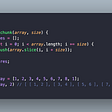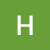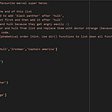# Solving Popular Algorithms: Self Dividing Numbers

`[1,2,3,4,5,6,7,8,9,11,12,15,22]`
`selfDividingNumbers = (left, right) => {   let allNums = []      for (let i = left; i <= right; i++) {      allNums.push(i)   }}`
`selfDividingNumbers = (left, right) => {   //step one: creating an array of all possible numbers   let allNums = []      for (let i = left; i <= right; i++) {      allNums.push(i)   }   //step two: creating an object of all possible numbers   //plus their digits    let numsObj = {}      for (let j = 0; j < allNums.length; j++) {      numsObj[allNums[j]] = allNums[j].toString().split("")   }}`
`selfDividingNumbers = (left, right) => {   //step one: creating an array of all possible numbers   let allNums = []      for (let i = left; i <= right; i++) {      allNums.push(i)   }   //step two: creating an object of all possible numbers   //plus their digits    let numsObj = {}      for (let j = 0; j < allNums.length; j++) {      numsObj[allNums[j]] = allNums[j].toString().split("")   }      //step three: looping through our objects keys and values   //to find what numbers are self dividing   for (let num in numsObj) {      for (let char of numsObj[num] {         if (parseInt(num) % parseInt(char) !== 0) {            delete numsObj[num]         }      }   }}`
`selfDividingNumbers = (left, right) => {   //step one: creating an array of all possible numbers   let allNums = []      for (let i = left; i <= right; i++) {      allNums.push(i)   }//step two: creating an object of all possible numbers   //plus their digits    let numsObj = {}      for (let j = 0; j < allNums.length; j++) {      numsObj[allNums[j]] = allNums[j].toString().split("")   }      //step three: looping through our objects keys and values   //to find what numbers are self dividing   for (let num in numsObj) {      for (let char of numsObj[num] {         if (parseInt(num) % parseInt(char) !== 0) {            delete numsObj[num]         }      }   }      //NEVER FORGET TO RETURN!!!   return Object.keys(numsObj).map(num => parseInt(num))}`

--

--

--

## More from HopeGiometti

Love podcasts or audiobooks? Learn on the go with our new app.

## Can React Hooks useReducer and useContext replace Redux?## Day 32 | 100 Days Full-Stack Challenge## Full isomorphic create-react-app + SSR + npm packed shared components with CSS## Image classification from Amazon SageMaker to your phone with TensorFlowJS## A Vim-based world, WebSummit@Lisbon, and a LOT of JavaScript 👇## Day 39 | 100 Days Full-Stack Challenge## Map, Filter and Reduce in Python## ARRAYS AND STRINGS DATA STRUCTURES.